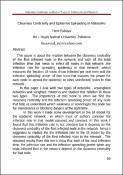## Closeness Centrality and Epidemic Spreading in Networks

 dc.contributor.author Fares Rabaya' dc.date.accessioned 2017-05-03T09:37:01Z dc.date.available 2017-05-03T09:37:01Z dc.date.issued 2010-08-02 dc.description.abstract

This paper is about the relation between the closeness centrality of the first infected node in the network and each of the total infection time that needs to infect all nodes in that network ,the infection rate for spreading epidemics in that network ,which measures the fraction of nodes those infected per unit time and the infection spreading power of that node,that masures the power for each node to spread the epidemic to other uninfected nodes in that network .
In this paper I deal with two types of networks , unweighted networks and weighted networks and studied that relation in those two types . The importance of this work is when we find the closeness centrality and the infection spreading power of any node that help us understand which weakness or advantages this node has for maintenance or blocking dangers at the right time .
In this work I made some development in the SI model for the epidemic network in which most of authors consider the infection rate in that model assumed and constant. In this work I found that this infection rate is not constant but it depends on the closeness centrality of the first infected node in the network ,hence I suggested to replace the the infection rate in the SI model by the closeness centrality of the first infected node in the network . The obtained results from this work show that each of the total infection time, the infection rate and the infection spreading power when any node infected first in the network depend on the closeness centrality for that node .

en dc.description.abstract

This paper is about the relation between the closeness centrality of the first infected node in the network and each of the total infection time that needs to infect all nodes in that network ,the infection rate for spreading epidemics in that network ,which measures the fraction of nodes those infected per unit time and the infection spreading power of that node,that masures the power for each node to spread the epidemic to other uninfected nodes in that network .
In this paper I deal with two types of networks , unweighted networks and weighted networks and studied that relation in those two types . The importance of this work is when we find the closeness centrality and the infection spreading power of any node that help us understand which weakness or advantages this node has for maintenance or blocking dangers at the right time .
In this work I made some development in the SI model for the epidemic network in which most of authors consider the infection rate in that model assumed and constant. In this work I found that this infection rate is not constant but it depends on the closeness centrality of the first infected node in the network ,hence I suggested to replace the the infection rate in the SI model by the closeness centrality of the first infected node in the network . The obtained results from this work show that each of the total infection time, the infection rate and the infection spreading power when any node infected first in the network depend on the closeness centrality for that node .

ar dc.identifier.uri https://hdl.handle.net/20.500.11888/9560 dc.title Closeness Centrality and Epidemic Spreading in Networks en dc.title Closeness Centrality and Epidemic Spreading in Networks ar dc.type Other
##### Original bundle
Now showing 1 - 1 of 1